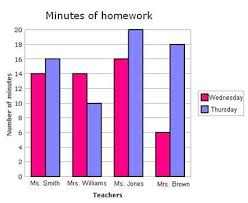# Graphing Project - 6th Math

6th Grade Math - Graphing Project

Due Date: Friday, March 26th, 2021

Each graph examined in the chapter has a different purpose.  Students are to read through the description of each type of graph.  The students then need to determine four different types of data to graph and collect the appropriate data.  The meanmedianmode, and range for each will need to be calculated for three of the graphs.  Print an appropriate graph all data sets.  A spreadsheet, the websites given, or graphing by (use a straightedge!) hand are all appropriate ways to display the data.  Each graph needs to have a title and be properly labeled.  Give credit for each graph of how the data was (used a website, took a survey, sports section, etc.) collected. Submit the four graphs, data and necessary calculations when the project is complete.  This is a test grade.

A useful Website:  http://nces.ed.gov/nceskids

Another useful website, http://www.shodor.org/interactivate/activities/

Students may use other websites that create graphs, but these two have been presented in class.

Bar Graph – A bar graph uses vertical or horizontal bars to display numerical information. It us used primarily to compare amounts.

Student work:  Determine a project where data will be used in comparison and record such data.  Example:  The winning scores in five basketball games.  Graph the data using one of three methods:  a spreadsheet, the website given above or an equivalent (be sure to credit the source) or graph the data by hand using a straightedge and colored pencils.  Calculate the Mean, Median, Mode, and Range for the data collected.

Broken Line Graph – uses a series of segments to show changes in data.  Usually a line graph shows trends or changes over time.

Student work:  Collect a different set of data where the data is set against a timeframe or a trend needs to be determined.  Example:  Amount of mail that arrives each day.  Graph the data using one of three methods:  a spreadsheet, the website given above or an equivalent (be sure to credit the source) or graph the data by hand using a straightedge and colored pencils.  Calculate the Mean, Median, Mode, and Range for the data collected.

Circle Graph – is a graph of data where the entire circle represents the whole.  Each wedge represents a part of the whole.

Student work:  Collect a third data set to be used in comparison to the whole and record such data.  Example:  Graph the activities which typically make up a student’s day.  Graph the data using one of three methods:  a spreadsheet, the website given above or an equivalent (be sure to credit the source) or graph the data by hand using a straightedge and colored pencils.  Calculate the Mean, Median, Mode, and Range for the data collected.

Histogram – is a bar graph that shows the frequency of each data item.  Histograms use equal-sized intervals to display the data.

Student work:  Determine a project where data will be used in comparison and record such data.  Example:  The number of products people buy in a store.  This may need to be graphed by hand, but there is at least one website that can graph histograms.  The Mean, Median, Mode, and Range do not need to be calculated for this graph.

Assemble all four graphs and the three sets of mean, median, mode, and range submit the package on or before the due date.

Include the data lists used to make the graphs.